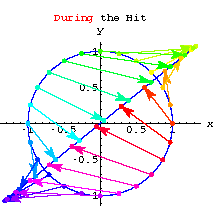Introduction
Matrix Action
Perpframes,
Aligners and Hangers
Stretchers
Coordinates
Projections
SVD
Matrix Subspaces
Linear Systems
Pseudo-Inverse
Condition Number
Matrix Norm
Rank One
Image Compression
Noise Filtering
Matrix Hits
2D Action

# Matrix Action

3D Action
Exercises

## Matrix Hits

When you hit a point with a matrix you get another point.

When the matrix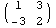hits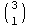you get### Two different ways to compute a matrix times a point.

COLUMN WAY to compute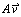: Take a linear combination of the COLUMNS of A using the weights from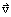. E.g.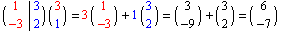ROW WAY to compute: Dot each ROW of A withE.g.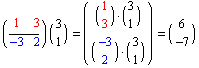## 2D Matrix Action

The plot below shows some color coded points on the unit circle. Move your mouse over the plot to see what happens when the matrixhits the points on the unit circle.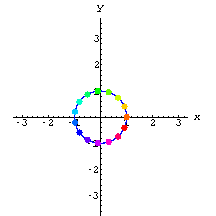You can see that the matrix stretches and rotates the unit circle--that's Matrix Action.

### Check out a few more.

Put your cursor over a matrix to see the matrix hit the unit circle.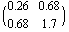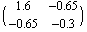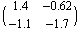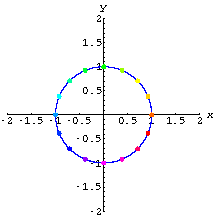### You can hit other curves too.

Check out the same three matrices hitting a damped sine wave.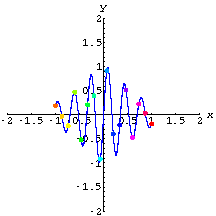## 3D Matrix Action

If you have a 3 by 3 matrix you can hit 3D surfaces.

Check out the matrix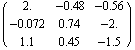hitting this surface.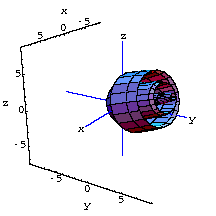The matrix appears to be rotating and stretching the surface. That's 3D matrix action.

## Exercises

 The plot to the right shows a matrix A hitting the unit circle.  Give estimates for A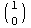and A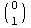. Give estimates for two different points that A sends to {0,0}.